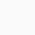# Fun with Prime Numbers: The Mysterious World of Mathematics

Learn about prime numbers, solve math problems and explore recent discoveries and theories.

Prime numbers are one of the most important subjects in mathematics. Many mathematicians from ancient times to the 21st century have studied prime numbers.

In this math course, you will learn the definition and basic properties of prime numbers, and how they obey mysterious laws. Some prime numbers were discovered several hundred years ago whereas others have only been proven recently. Even today, many mathematicians are trying to discover new laws of prime numbers.

Calculating with a pen and paper, you will explore the mysterious world of prime numbers. Join us as we tackle math problems, and work together to discover new laws on prime numbers. Let’s study and have fun!

• The definition and basic properties of prime numbers
• Laws of prime numbers
• Math problems with prime numbers
• Recent progress made around prime numbers
• Polynomial analogues of prime numbers
Fun with Prime Numbers: The Mysterious World of Mathematics

1

We will be happy to hear your thoughts